# Engineering Maths - Probability

>>>>>>>>Probability

• A

3/13• B

4/13• C

4/17• D

5/13• Option : B
• Explanation : Probability of drawing an ace from a deck of
52 cards =  4/52
Similarly the probability of drawing a card of
Probability of drawing an ace of spades = 1/52
Since two events (i.e. a card being an ace and a
card being of spades) are not mutually exclusive.
therefore
Probability of drawing an ace or a spade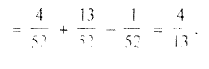• A

True• B

False• Option : A
• Explanation :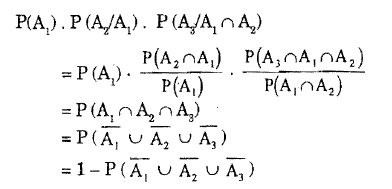• A

P(A) ≥ P(B)• B

P(A) ≤ P(B)• C

P(A) < P(B)• D

P(A) > P(B)• Option : A
• Explanation :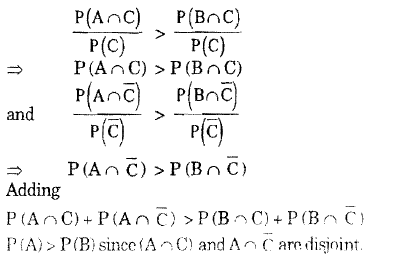• A

True• B

False• Option : A
• Explanation :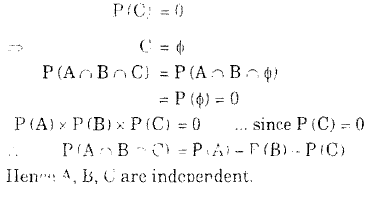• A

1/4• B

3/4• C

4/5• D

1/7• Option : B
• Explanation :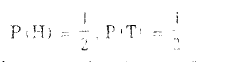Let A be the event of getting 5 or 6
P(A) = 1/3 and B be the event of getting 1,2,3,4
P(B) = 2/3
In order to get a 'Head' before "5 or 6",  the
following events have to occur:
H, TBH, TBTBH, TBTBTBH, ....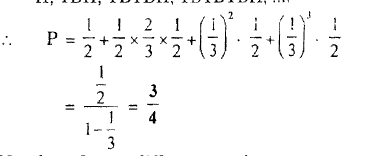Related Quiz.
Probability